xValidityOverview

In logic
Logic
In philosophy, Logic is the formal systematic study of the principles of valid inference and correct reasoning. Logic is used in most intellectual activities, but is studied primarily in the disciplines of philosophy, mathematics, semantics, and computer science...

, argument
Argument
In philosophy and logic, an argument is an attempt to persuade someone of something, or give evidence or reasons for accepting a particular conclusion.Argument may also refer to:-Mathematics and computer science:...

is valid if and only if its conclusion is entailed
Entailment
In logic, entailment is a relation between a set of sentences and a sentence. Let Γ be a set of one or more sentences; let S1 be the conjunction of the elements of Γ, and let S2 be a sentence: then, Γ entails S2 if and only if S1 and not-S2 are logically inconsistent...

by its premises, a formula
Well-formed formula
In mathematical logic, a well-formed formula, shortly wff, often simply formula, is a word which is part of a formal language...

is valid if and only if it is true under every interpretation
Interpretation (logic)
An interpretation is an assignment of meaning to the symbols of a formal language. Many formal languages used in mathematics, logic, and theoretical computer science are defined in solely syntactic terms, and as such do not have any meaning until they are given some interpretation...

, and an argument form (or schema) is valid if and only if every argument of that logical form
Logical form
In logic, the logical form of a sentence or set of sentences is the form obtained by abstracting from the subject matter of its content terms or by regarding the content terms as mere placeholders or blanks on a form...

is valid.
An argument is valid if and only if the truth of its premises entails
Entailment
In logic, entailment is a relation between a set of sentences and a sentence. Let Γ be a set of one or more sentences; let S1 be the conjunction of the elements of Γ, and let S2 be a sentence: then, Γ entails S2 if and only if S1 and not-S2 are logically inconsistent...

the truth of its conclusion. It would be self-contradictory to affirm the premises and deny the conclusion.Encyclopedia
In logic
Logic
In philosophy, Logic is the formal systematic study of the principles of valid inference and correct reasoning. Logic is used in most intellectual activities, but is studied primarily in the disciplines of philosophy, mathematics, semantics, and computer science...

, argument
Argument
In philosophy and logic, an argument is an attempt to persuade someone of something, or give evidence or reasons for accepting a particular conclusion.Argument may also refer to:-Mathematics and computer science:...

is valid if and only if its conclusion is entailed
Entailment
In logic, entailment is a relation between a set of sentences and a sentence. Let Γ be a set of one or more sentences; let S1 be the conjunction of the elements of Γ, and let S2 be a sentence: then, Γ entails S2 if and only if S1 and not-S2 are logically inconsistent...

by its premises, a formula
Well-formed formula
In mathematical logic, a well-formed formula, shortly wff, often simply formula, is a word which is part of a formal language...

is valid if and only if it is true under every interpretation
Interpretation (logic)
An interpretation is an assignment of meaning to the symbols of a formal language. Many formal languages used in mathematics, logic, and theoretical computer science are defined in solely syntactic terms, and as such do not have any meaning until they are given some interpretation...

, and an argument form (or schema) is valid if and only if every argument of that logical form
Logical form
In logic, the logical form of a sentence or set of sentences is the form obtained by abstracting from the subject matter of its content terms or by regarding the content terms as mere placeholders or blanks on a form...

is valid.

## Validity of arguments

An argument is valid if and only if the truth of its premises entails
Entailment
In logic, entailment is a relation between a set of sentences and a sentence. Let Γ be a set of one or more sentences; let S1 be the conjunction of the elements of Γ, and let S2 be a sentence: then, Γ entails S2 if and only if S1 and not-S2 are logically inconsistent...

the truth of its conclusion. It would be self-contradictory to affirm the premises and deny the conclusion. The corresponding conditional of a valid argument is a logical truth
Logical truth
Logical truth is one of the most fundamental concepts in logic, and there are different theories on its nature. A logical truth is a statement which is true and remains true under all reinterpretations of its components other than its logical constants. It is a type of analytic statement.Logical...

and the negation of its corresponding conditional is a contradiction
In classical logic, a contradiction consists of a logical incompatibility between two or more propositions. It occurs when the propositions, taken together, yield two conclusions which form the logical, usually opposite inversions of each other...

. The conclusion is a logical consequence of its premises.

An argument that is not valid is said to be “invalid”.

An example of a valid argument is given by the following well-known syllogism
Syllogism
A syllogism is a kind of logical argument in which one proposition is inferred from two or more others of a certain form...

(also known as modus ponens
Modus ponens
In classical logic, modus ponendo ponens or implication elimination is a valid, simple argument form. It is related to another valid form of argument, modus tollens. Both Modus Ponens and Modus Tollens can be mistakenly used when proving arguments...

):
All men are mortal.
Socrates is a man.
Therefore, Socrates is mortal.

What makes this a valid argument is not that it has true premises and a true conclusion, but the logical necessity of the conclusion, given the two premises. The argument would be just as valid were the premises and conclusion false. The following argument is of the same logical form
Logical form
In logic, the logical form of a sentence or set of sentences is the form obtained by abstracting from the subject matter of its content terms or by regarding the content terms as mere placeholders or blanks on a form...

but with false premises and a false conclusion, and it is equally valid:
All cups are green.
Socrates is a cup.
Therefore, Socrates is green.

No matter how the universe might be constructed, it could never be the case that these arguments should turn out to have simultaneously true premises but a false conclusion. The above arguments may be contrasted with the following invalid one:
All men are mortal.
Socrates is mortal.
Therefore, Socrates is a man.

In this case, the conclusion does not follow inescapably from the premises. All men are mortal, but not all mortals are men. Every living creature is mortal; therefore, even though both premises are true and the conclusion happens to be true in this instance, the argument is invalid.

A standard view is that whether an argument is valid is a matter of the argument’s logical form
Logical form
In logic, the logical form of a sentence or set of sentences is the form obtained by abstracting from the subject matter of its content terms or by regarding the content terms as mere placeholders or blanks on a form...

. Many techniques are employed by logicians to represent an argument’s logical form. A simple example, applied to two of the above illustrations, is the following: Let the letters ‘P’, ‘Q’, and ‘S’ stand, respectively, for the set of men, the set of mortals, and Socrates. Using these symbols, the first argument may be abbreviated as:
All P are Q.
S is a P.
Therefore, S is a Q.

Similarly, the third argument becomes:
All P are Q.
S is a Q.
Therefore, S is a P.

An argument is formally valid if its form is one such that for each interpretation under which the premises are all true, the conclusion is also true.
As already seen, the interpretation given above does cause the second argument form to have true premises and false conclusion, hence demonstrating its invalidity.

## Valid formula

A formula of a formal language
Formal language
A formal language is a set of words—that is, finite strings of letters, symbols, or tokens that are defined in the language. The set from which these letters are taken is the alphabet over which the language is defined. A formal language is often defined by means of a formal grammar...

is a valid formula if and only if it is true under every possible interpretation of the language.

## Validity of statements

A statement can be called valid, i.e. logical truth, if it is true in all interpretations.

For example: If no god is mortal
Human
Humans are the only living species in the Homo genus...

, then no mortal is a god.

In logical form, this is:
If no P is Q, then no Q is P.

A given statement may be entailed by other statements, i.e. the given statement must be true if the other statements are true. This means that an argument with the given statement as its conclusion and the other statements as its premises is a valid argument. The corresponding conditional of a valid argument is a logical truth.

## Validity and soundness

Validity of deduction is not affected by the truth of the premise or the truth of the conclusion. The following deduction is perfectly valid:
All fire-breathing rabbits live on Mars
All humans are fire-breathing rabbits
Therefore all humans live on Mars

The problem with the argument is that it is not sound
Soundness
In mathematical logic, a logical system has the soundness property if and only if its inference rules prove only formulas that are valid with respect to its semantics. In most cases, this comes down to its rules having the property of preserving truth, but this is not the case in general. The word...

. In order for a deductive argument to be sound, the deduction must be valid and all the premises true.

## Satisfiability and validity

Model theory
Model theory
In mathematics, model theory is the study of mathematical structures using tools from mathematical logic....

analyzes formulae with respect to particular classes of interpretation in suitable mathematical structures. On this reading, formula is valid if all such interpretations make it true. An inference is valid if all interpretations that validate the premises validate the conclusion. This is known as semantic validity.

## Preservation

In truth-preserving validity, the interpretation under which all variables are assigned a truth value of 'true' produces a truth value of 'true'.

In a false-preserving validity, the interpretation under which all variables are assigned a truth value of ‘false’ produces a truth value of ‘false'.
Preservation properties Logical connective
Logical connective
In logic, a logical connective is a symbol or word used to connect two or more sentences in a grammatically valid way, such that the compound sentence produced has a truth value dependent on the respective truth values of the original sentences.Each logical connective can be expressed as a...

sentences
True and false preserving: Logical conjunction (AND,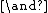)
Logical conjunction
In logic and mathematics, a two-place logical operator and, also known as logical conjunction, results in true if both of its operands are true, otherwise the value of false....

Logical disjunction (OR,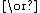)
Logical disjunction
In logic and mathematics, a two-place logical connective or, is a logical disjunction, also known as inclusive disjunction or alternation, that results in true whenever one or more of its operands are true. E.g. in this context, "A or B" is true if A is true, or if B is true, or if both A and B are...

True preserving only: Tautology (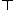)
Tautology (logic)
In logic, a tautology is a formula which is true in every possible interpretation. Philosopher Ludwig Wittgenstein first applied the term to redundancies of propositional logic in 1921; it had been used earlier to refer to rhetorical tautologies, and continues to be used in that alternate sense...

Biconditional (XNOR,)
Logical biconditional
In logic and mathematics, the logical biconditional is the logical connective of two statements asserting "p if and only if q", where q is a hypothesis and p is a conclusion...

Implication (Converse implication ()
Converse implication
Converse implication is the converse of implication. That is to say; that for any two propositions P and Q, if Q implies P, then P is the converse implication of Q.It may take the following forms:-Truth table:The truth table of A⊂B-Venn diagram:...

False preserving only: Contradiction (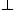)
In classical logic, a contradiction consists of a logical incompatibility between two or more propositions. It occurs when the propositions, taken together, yield two conclusions which form the logical, usually opposite inversions of each other...

Exclusive disjunction (XOR,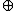Nonimplication (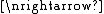)
Material nonimplication
Material nonimplication or abjunction is the negation of implication. That is to say that for any two propositions P and Q, if P does not imply Q, then P is the material nonimplication of Q....

Converse nonimplication (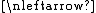)
Non-preserving: Proposition  Negation ()
Negation
In logic and mathematics, negation, also called logical complement, is an operation on propositions, truth values, or semantic values more generally. Intuitively, the negation of a proposition is true when that proposition is false, and vice versa. In classical logic negation is normally identified...

Alternative denial (NAND,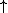)
Sheffer stroke
In Boolean functions and propositional calculus, the Sheffer stroke, named after Henry M. Sheffer, written "|" , "Dpq", or "↑", denotes a logical operation that is equivalent to the negation of the conjunction operation, expressed in ordinary language as "not both"...

Joint denial (NOR,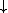)
Logical NOR
In boolean logic, logical nor or joint denial is a truth-functional operator which produces a result that is the negation of logical or. That is, a sentence of the form is true precisely when neither p nor q is true—i.e. when both of p and q are false...

## n-Validity

A formula A of a first order language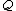is n-valid iff
If and only if
In logic and related fields such as mathematics and philosophy, if and only if is a biconditional logical connective between statements....

it is true for every interpretation ofthat has a domain
Domain (mathematics)
In mathematics, the domain of definition or simply the domain of a function is the set of "input" or argument values for which the function is defined...

of exactly n members.

### ω-Validity

A formula of a first order language is ω-valid iff
If and only if
In logic and related fields such as mathematics and philosophy, if and only if is a biconditional logical connective between statements....

it is true for every interpretation of the language and it has a domain
Domain (mathematics)
In mathematics, the domain of definition or simply the domain of a function is the set of "input" or argument values for which the function is defined...

with an infinite
Countable set
In mathematics, a countable set is a set with the same cardinality as some subset of the set of natural numbers. A set that is not countable is called uncountable. The term was originated by Georg Cantor...

number of members.